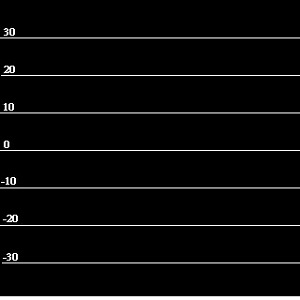# Enter numbers in the coordinate axis

How can I get the numbers on the coordinate axis?

Your question is not clear, can you please elaborate on what your project should do and what information you need from what component?

I want to show the numbers on the graph drawing page that is unified.

Take a look at some of these exercises made by @Juan_Antonio. I think you can adapt one of those to do what you need. Other than that, the canvas is just a white empty “canvas” or “paper” where you have to draw whatever you need. There’s no pre made graphics like the one you posted.

2 Likes

I saw this but written in Java I want to have this in appinventor.

Here a shots from an AI app using a Canvas and all native Blocks. Is this what you want to do with AI?

The graphing screen auto scales both x and y axis based on the dataset, input as a List.
The Canvas can also be used with a set Canvas background that can be used as the coordinate scale for data sets that have y values between 30 and -30.What have you tried Fatemeh? You need a Canvas and math to convert World coordinates to Screen coordinates:

Posted is an image of the algorithm I use to convert the map x values to a screen coordinate (mapW2SxLin). Do that for the y values too.

The two functions are used to convert your plot data x and y values using AI2 block procedures. The other two functions perform the reverse and you should not need to do that.

The algorithms to convert World Coordinates to the Screen coordinates of the Canvas control were derived from Pascal code here: http://www.ibrtses.com/delphi/dmcs.html

and is summarized:

Mapping world and screen coordinate systems to each other is
done by scaling with respect to an offset.

be the world window be defined as:

[xLow…xHigh,yLow…yHigh], the coordinates are of type float

be the screen window be defined as:

[tlx…brx,tly…bry], the coordinates are of type integer

the following function do the conversion :

function mapW2SxLin(xf:float):integer;
begin
result:=round(tlx+(xf-xlow)*(brx-tlx)/(xhigh-xlow));
end;

function mapW2SyLin(yf:float):integer;
begin
result:=round(bry-(yf-ylow)*(bry-tly)/(yhigh-ylow));
end;

function mapS2WxLin(xs:integer):float;
begin
result:=xlow+(xs-tlx)*(xhigh-xlow)/(brx-tlx);
end;

function mapS2WyLin(ys:integer):float;
begin
result:=yhigh-(ys-tly)*(yhigh-ylow)/(bry-tly);
end;

Helpful is this article from Chapter 17. Creating Animated Apps of App Inventor 2: Create your own Android Apps by Wolber, Abelson et al.

Something similar could also be done with Blocks using different scaling techniques.

Regards,
Steve

1 Like